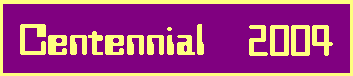# 3-Ring Class Fields

## 1. Galois Correspondence.

Arnold Scholz uses the following notation 
for S3-fields and their automorphism groups:

Indices of subfields denote their absolute degrees
over the rational number field P (capital Rho instead of Q).
The generating automorphisms satisfy the relations
T2 = E, S3 = E, TS = S2T,
where E denotes the identity automorphism.

The following diagram summarizes the Galois correspondence:

 K6 / | K3 | | K2 | / P
 Gal(K6|K6) = < E > / | Gal(K6|K3) = < T > | | Gal(K6|K2) = < S > | / Gal(K6|P) = < S,T >

The discriminants are connected by the relations
D3 = f2D2 (Hasse)
and D6 = D23(f2)2 (Hilbert)
= D2D32,
whence K6 is a 3-ring class field
with conductor f over the quadratic base field K2.

## 2. Expansion of Old Units.

According to Dirichlet ,
the torsion free unit groups of K2, K3, K6
are of the following shape, depending on the signatures:

in the complex case, D2 < 0,
U2 = < 1 >, U3 = < e3 >,
U6 = < e6, e6S >

and in the totally real case, D2 > 0,
U2 = < e >, U3 = < e1, e2 >,
U6 = < e, eta1, eta2, eta1S, eta2S >

In general, the units of K6
cannot be completely generated by
the units of all subfields and their conjugates,
which are called old units by Arnold Scholz:
Uo = U2*U3*U3S, and in particular
Uo = < e3, e3S > for D2 < 0,
and Uo = < e, e1, e2, e1S, e2S > for D2 > 0.

u = (U6:Uo) is called the index of old units
and may take the values
1,3 for D2 < 0,
and 1,3,9 for D2 > 0.

The regulators of U2, U3, Uo, U6
are connected by the following relations:
Ro = 3*R2R32 with R2 = 1 for D2 < 0,
and Ro = 9*R2R32 for D2 > 0
is the volume of the coarse lattice mesh of the
old unit group Uo in logarithmic space,
and for both signatures we have
R6 = (1 / u)*Ro
as the volume of the finer lattice mesh of the
full unit group U6 in logarithmic space.

Of course, the smaller regulator R6 <= Ro
corresponds to an expansion of the old units U6 >= Uo.

## 3. Contraction of Ideal Class Groups.

Using the analytical class number formula,
Arnold Scholz derived the following class number relations
from the regulator formulas:
h6 = (u / 3)*h2h32 for D2 < 0,
and h6 = (u / 9)*h2h32 for D2 > 0.

According to the index u of old units
and the representation of units with Hilbert's theorem 90,
Scholz distinguishes several types of
cyclic cubic relative extensions K6|K2,
which are simply coarse variants of the principal factorization types:

In the complex case, we have 2 types:
Type ALPHA <==> u = 1
<==> e3 = e6
<==> h6 = (1 / 3)*h2h32
Type BETA/GAMMA <==> u = 3
<==> e3 = e6S-1
<==> h6 = h2h32

For Type BETA, resp. GAMMA,
Norm6|2(e6) = e61+S+S2 = 1, resp. zeta,
a primitive third root of unity.

And in the totally real case, we have 3 types:
Type ALPHA <==> u = 1
<==> e1 = eta1 and e2 = eta2
<==> h6 = (1 / 9)*h2h32
Type BETA/DELTA <==> u = 3
<==> e1 = eta1 but e2 = eta2S-1
<==> h6 = (1 / 3)*h2h32
Type GAMMA/EPSILON <==> u = 9
<==> e1 = eta1S-1 and e2 = eta2S-1
<==> h6 = h2h32

For Type ALPHA/BETA/GAMMA, resp. DELTA/EPSILON,
Norm6|2(eta2) = eta21+S+S2 = 1, resp. e,
the fundamental unit of K2,
whereas always Norm6|2(eta1) = eta11+S+S2 = 1.

Arnold Scholz gives an interpretation of these results:
wheras for all primes p != 3, the p-class group of K6 is a direct product
C6 = C2*C3*C3S,
we have a contraction of the 3-class groups for the
complex type ALPHA end the totally real types ALPHA, BETA, DELTA,
since certainly C2 is contained in C3*C3S
and possibly C3 and C3S have a non-trivial intersection.

## 4. The Galois Group of (K6)1 over K2.

This will be the topic of our next article.
 References:  Arnold Scholz, Idealklassen und Einheiten in kubischen Körpern, Monatsh. Math. Phys. 40 (1933), 211 - 222

 <| Navigation Center <| <| Back to Algebra <|